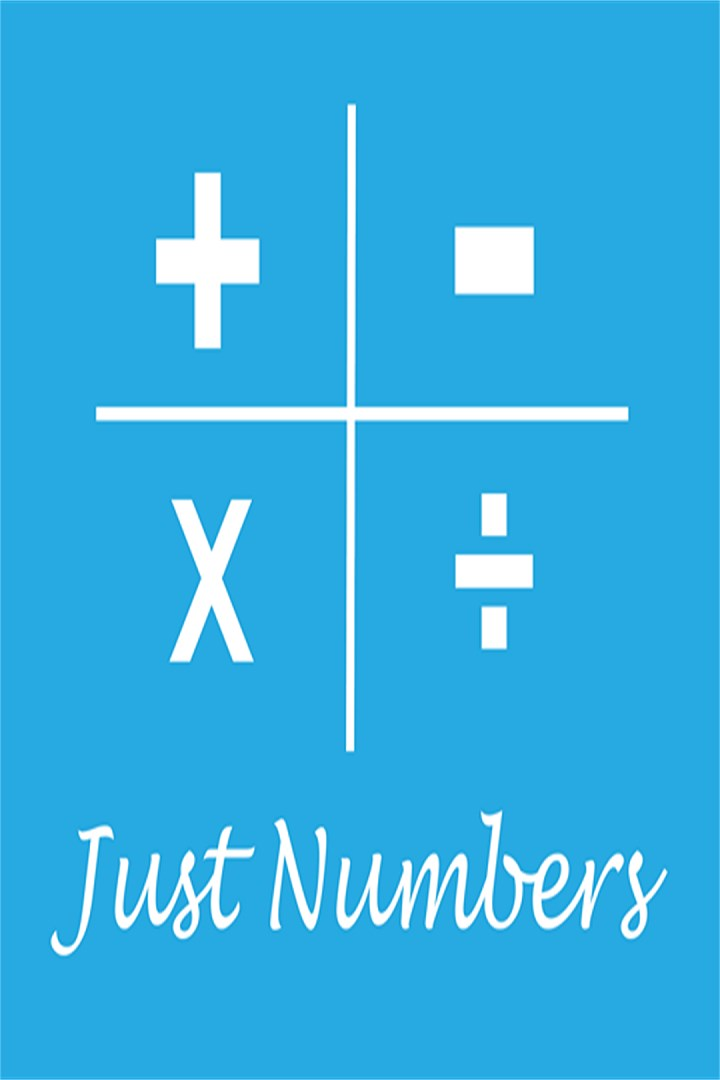Free
Overview System Requirements

## Description

Math Game to practice addition, subtraction, multiplication or division. This game is designed to test your mental math and problem-solving skills while having fun! Choose from the following operators and set your desired level to start playing: ► Addition (+) ► Subtraction (-) ► Multiplication (X) ► Division (÷) Each operator has 6 game levels: ► Level 1 – 25 Math Problems ► Level 2 – 50 Math Problems ► Level 3 – 75 Math Problems ► Level 4 – 100 Math Problems ► Word Problem – 10 Math Problems ► Random Numbers – 25 Math Problems Note: Level 1 to 4 and Word problems are set with pre-defined math problems, this is designed as math worksheet to reinforce your knowledge of calculating with multi-digit whole numbers, solving simple math problems and understanding multiplication and division. How to Play: ► Answer can be entered through the Numeric Keypad ► User input required to enable the "Submit Button", for the users to submit their answer. ► On submission of each answer, question are refreshed until the game level is completed. FEATURES: ► Card values can range from 0-1000 ► Each correct answer earn one point ► Correct Answer displayed in the event wrong answer entered ► At the end of each game, score displayed to help motivate the gamer to practice and improve ► Train endurance by solving as much tasks as you want without time limits ► Helps keep you sharp Thank you for your interest and downloading the game and appreciate your valuable feedback / review.

## Features

• Random Subtraction
• Random Multiplication
• Random Division
• Whole Numbers
• Math Challenge
• Pre-defined math problems

Transpose Solutions

Transpose Solutions

#### Developed by

Transpose Solutions

10/16/2018

19.3 MB

For all ages

Educational

#### This app can

Access your Internet connection

#### Installation

Get this app while signed in to your Microsoft account and install on up to ten Windows 10 devices.

#### Language supported

English (United States)